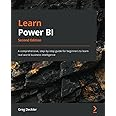cancel
Showing results for
Did you mean:## Look up a value of a cell in the same column and using it in a DAX formula

Good Day, I have been struggling with transforming an Excel workbook into Power BI Desktop. The custom column is "Pop. per Density", and its an animal "Population" divided by a denominator. That denominator is the Median of the "Pop. Density" if the animal in the row matches the the animals in the "Animal" column.

Excel Formula:

Pop. per Density = IFERROR([@Population]/MEDIAN(IF([Animal]=[@Animal],[Pop. Density],"")),"")

I am still a novice with DAX, but in Excel the formula is straight forward. I just have no idea on how to reference a cell in a column, in PBI, and I cant seem to find a troubleshoot on that either.

Pop. per DensityIF( ISERROR( SEARCH("Cat", 'Animals'[Animal]) ), 0, CONVERT(MEDIAN( 'Animals'[Pop. Density]), INTEGER))

I just want to replace that string "Cat" with the value of the cell. Am I close?

 Animal Population Pop. Density Pop. per Density Cat 2.97 Fox 4.51 29.02 0.15 Eagle 2.11 Snake 1.34 Dog 5.62 18.64 0.39 Owl 0.11 Dog 1.28 4.54 0.09 Monkey 1.17 Dog 7.62 14.30 0.53 Frog 12.45

Any help on the above would be most appreciated!

1 ACCEPTED SOLUTIONSuper User

@RiskyBiscuts

Can you see if the following calculated column solves your problem?

``````Pop. per Density =

var __ani = Animal[Animal ]
var __deno =
CALCULATE(
MEDIAN( Animal[Pop. Density] ),
Animal[Animal ] = __ani,
REMOVEFILTERS(Animal)
)
var __pop = Animal[Population ]
return
DIVIDE( __pop , __deno )``````Did I answer your question? Mark my post as a solution! and hit thumbs up
5 REPLIES 5Super User

@RiskyBiscuts

Can you see if the following calculated column solves your problem?

``````Pop. per Density =

var __ani = Animal[Animal ]
var __deno =
CALCULATE(
MEDIAN( Animal[Pop. Density] ),
Animal[Animal ] = __ani,
REMOVEFILTERS(Animal)
)
var __pop = Animal[Population ]
return
DIVIDE( __pop , __deno )``````Did I answer your question? Mark my post as a solution! and hit thumbs upSpot on! Yes this is what I meant to solve. Only thing I need to do is ignore the 0 and null values in the "Pop. per Density", as it turns I get a null value when 50% of the "Pop. Density" values are less then 0 or null. Do you know how I can ignore those 0 and null values?Super User

@RiskyBiscuts

If you want to get rid of the blank and zero lines then you will have to create another with filter as follows:

``New Animal = FILTER( Animal , Animal[Pop. per Density] <> BLANK() )``Or, you can create a measure as blanks are automatically hidden

``````Measure Pop. per Density =

var __ani = SELECTEDVALUE(Animal[Animal ])
var __deno =
CALCULATE(
MEDIAN( Animal[Pop. Density] ),
Animal[Animal ] = __ani,
REMOVEFILTERS(Animal)
)
var __pop = SELECTEDVALUE(Animal[Population ])
return
DIVIDE( __pop , __deno )``````Did I answer your question? Mark my post as a solution! and hit thumbs upSuper User

@RiskyBiscuts - I think what you want is:

``````Pop. per Density Column =
MEDIANX(FILTER('Animals',[Animal]=EARLIER('Animals'[Animal])),[Pop. Density])
``````

Also, you can find a lot of Excel to DAX translations here: Excel to DAX Translation - Microsoft Power BI Community

Become an expert!: Enterprise DNA
External Tools: MSHGQM
Latest book!:
Learn Power BI 2nd EditionHey! Thanks for that link, its going to definately help me out!AnnouncementsThe first Microsoft-sponsored Power Platform Conference is coming in September. 100+ speakers, 150+ sessions, and what's new and next for Power Platform.#### Check it Out!

Mark your calendars and join us on Thursday, June 30 at 11a PDT for a great session with Ted Pattison!#### The Power BI Community Show

Join us on June 20 at 11 am PDT when Kim Manis shares the latest on Azure Synapse analytics, the Microsoft Intelligent Data Platform, and notable Power BI Updates from Microsoft Build 2022.Top Solution Authors
Top Kudoed Authors
Users online (3,420)